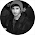## Sunday, July 8, 2012

### Color quantization

The aim of color clustering is to produce a small set of representative colors which captures the color properties of an image. Using the small set of color found by the clustering, a quantization process can be applied to the image to find a new version of the image that has been "simplified," both in colors and shapes.
In this post we will see how to use the K-Means algorithm to perform color clustering and how to apply the quantization. Let's see the code:
```from pylab import imread,imshow,figure,show,subplot
from numpy import reshape,uint8,flipud
from scipy.cluster.vq import kmeans,vq

# reshaping the pixels matrix
pixel = reshape(img,(img.shape*img.shape,3))

# performing the clustering
centroids,_ = kmeans(pixel,6) # six colors will be found
# quantization
qnt,_ = vq(pixel,centroids)

# reshaping the result of the quantization
centers_idx = reshape(qnt,(img.shape,img.shape))
clustered = centroids[centers_idx]

figure(1)
subplot(211)
imshow(flipud(img))
subplot(212)
imshow(flipud(clustered))
show()
```
The result shoud be as follows:

We have the original image on the top and the quantized version on the bottom. We can see that the image on the bottom has only six colors. Now, we can plot the colors found with the clustering in the RGB space with the following code:
```# visualizing the centroids into the RGB space
from mpl_toolkits.mplot3d import Axes3D
fig = figure(2)
ax = fig.gca(projection='3d')
ax.scatter(centroids[:,0],centroids[:,1],centroids[:,2],c=centroids/255.,s=100)

show()
```
And this is the result:

The result of the same script on another follows:

In this case I used four color. Here's the plot of the color in the RGB space:

1.I recently packaged this functionality into SimpleCV. You may want to take a look.

http://www.simplecv.org/docs/SimpleCV.html#i/SimpleCV.ImageClass.Image/palettize

2.Thank you Katherine, I never used SimpleCV.

3.Here is another implementation using the scikit-learn K-Means: http://scikit-learn.org/stable/auto_examples/cluster/plot_color_quantization.html

4.Do you normally compose for this blog or maybe for other Internet networks?

5.Hello Miss Teegans, I usually write only for this blog.

6.Can we get similar results using Agglomerative Clustering instead of K-means. If yes how can we proceed.

1.Hi Mehmood, the approach I followed is very simple. I thought the pixel as a sample. This way, every pixel is a point in the 3d space that you can cluster using any algorithm.

You scipy functions for agglomerative clustering are listed here: http://docs.scipy.org/doc/scipy/reference/cluster.hierarchy.html#module-scipy.cluster.hierarchy

7.I was able to make the script work, but I have two issues:
1) The input image is shown upside down.
2) I would like to tell Python to save the quantization result to a specified location on the hard drive.

Thanks in advance for the help

1.Hi Nazzareno, you can solve your first problem removing the call to flipup and the second using the function savefig (it's in pylab).

2.After those changes I get this error:
clustered.savefig('fig2.png')
AttributeError: 'numpy.ndarray' object has no attribute 'savefig'

I tried also to use the "from PIL import Image" and to save the resulting image with "clustered.save ('output.jpg')", but it gives me the same error. I'm an absolute noob :P what am I doing wrong?

3.savefig is a matplotlib function (check the documentation here: http://matplotlib.org/api/pyplot_api.html#matplotlib.pyplot.savefig). It saves the current image into a file.

4.I finally figured out what was the problem. The output from the clustered variable is not properly an image, but something similar to an array (I guess). So I needed to convert that variable into an actual image before being able to save it. I did so with:
output = Image.fromarray(clustered)

I don't know if it makes perfect sense, but in the end I'm now able to achieve what I was looking for :)
Thank you for the script and for the help.

8.Hi, I'm getting this error while running your code. Please have a look at the error message. Thanks in advance.

Traceback (most recent call last):
File "dominant.py", line 11, in
centroids,_ = kmeans(pixel,6) # six colors will be found
File "/Users/manisha.sangwan/src/scipy/scipy/cluster/vq.py", line 520, in kmeans
book, dist = _kmeans(obs, guess, thresh=thresh)
File "/Users/manisha.sangwan/src/scipy/scipy/cluster/vq.py", line 405, in _kmeans
code_book, has_members = _vq.update_cluster_means(obs, obs_code, nc)
File "_vq.pyx", line 345, in scipy.cluster._vq.update_cluster_means (scipy/cluster/_vq.c:4526)
TypeError: type other than float or double not supported

1.Hi mani, try to convert the values of the matrix pixel to float or double before using kmeans. Maybe the scipy developers updated the kmeans function adding a new type check.